# 中国疫情发展趋势预测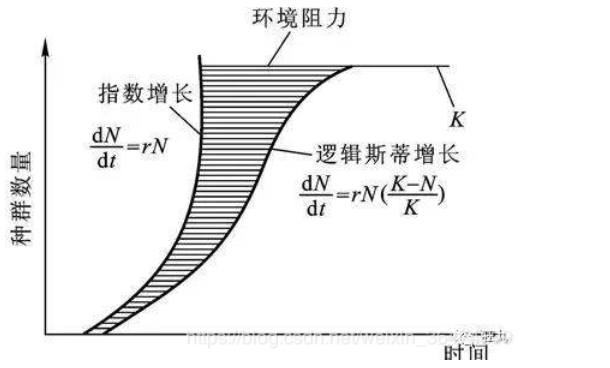logistic增长的曲线也称为s型曲线。下图左图为曲线数量，右图为增长速率。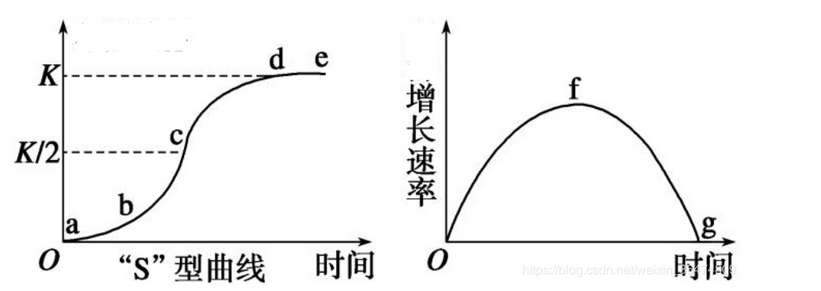• 开始期，由于种群个体数很少，密度增长缓慢，又称潜伏期。
• 加速期，随个体数增加，密度增长加快。
• 转折期，当个体数达到饱和密度一半（K/2),密度增长最快。
• 减速期，个体数超过密度一半（K/2)后，增长变慢。
• 饱和期，种群个体数达到K值而饱和。

import numpy as np
import matplotlib.pyplot as plt
import pandas as pd
import seaborn as sns
import warnings
# from scipy.optimize import curve_fit  # 非线性最小二乘法拟合
from scipy.optimize  import leastsq

warnings.filterwarnings('ignore')

plt.style.use("seaborn")
plt.rc('font', family='SimHei', size=13)  # 显示中文
plt.rcParams['axes.unicode_minus'] = False  # 用来正常显示负号

# 先读取数据
China_data

plt.plot(China_data['累计确诊'], label='确诊')
plt.plot(China_data['现存疑似'], label='现存疑似')
plt.plot(China_data['累计死亡'], label='死亡')
plt.plot(China_data['累计治愈'], label='治愈')
plt.legend(loc="best")
plt.xlabel("时间")
plt.title("2019-nCoV疫情曲线")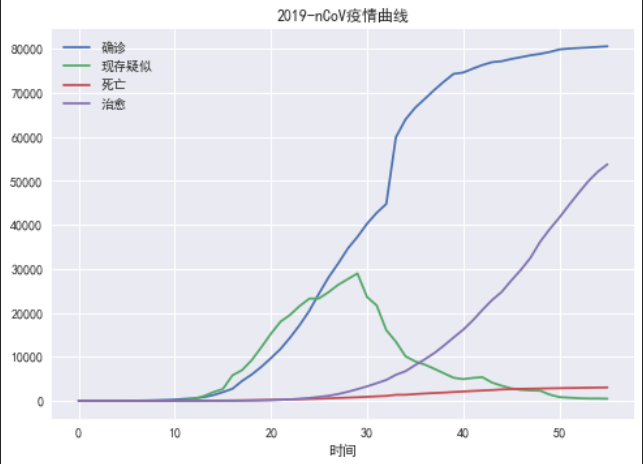# 逻辑斯蒂增长模型
def logistic_increase_function(p, t):
K, a, b = p
exp_value = np.exp(-a * (t - b))
return K / (1 + exp_value)
# 定义预测误差函数
def err_f(p, t, y):
return logistic_increase_function(p, t) - y
# 参数初始值
logistic_p0 = [80000, 0.8, 20]  # 初始值只要不是太离谱，最终都会收敛

# 我们选择的数据
t = np.array([i + 1 for i in range(56)])
China_y = China_data['累计确诊'].values

# 利用最小二乘法求解参数
logistic_params = leastsq(err_f, logistic_p0, args=(t, China_y))

China_p = logistic_params

# 利用我们定义的逻辑斯蒂增长函数预测
China_predict_data = logistic_increase_function(China_p, t)
China_predict_data

# 预测的误差
China_e = China_y - China_predict_data
China_e

# 绘制误差的散点图
plt.scatter(China_y, China_e)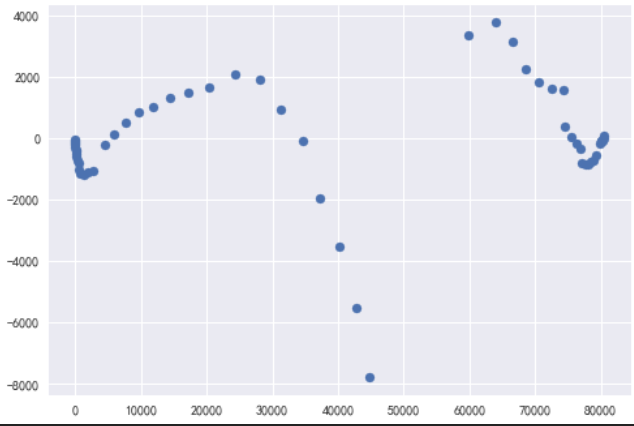# 绘图
plt.scatter(t, China_y, label="实际确诊患者数量")
plt.plot(t, China_predict_data, label='预测患者数量曲线')
plt.xlabel('time')
plt.ylabel('患者数量')
plt.legend(loc='best')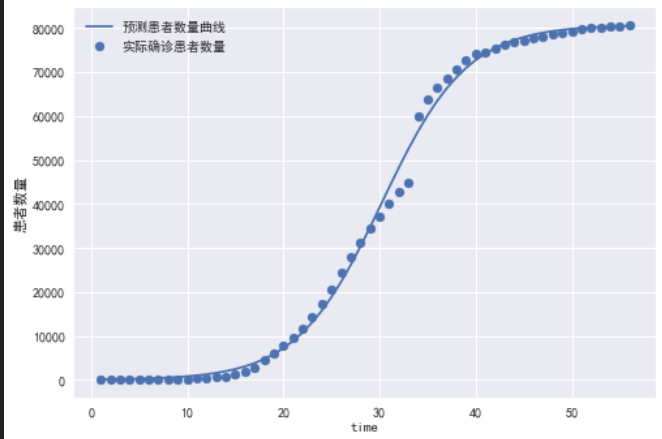# 预测中国疫情未来走势
future_t = [i + 1 for i in range(0, 100)]
China_future_fit = logistic_increase_function(China_p, future_t)
China_future_fit
# 绘图
plt.scatter(t, China_y, label="实际确诊患者数量")
plt.plot(future_t, China_future_fit, label="预测未来患者数量")
plt.xlabel('time')
plt.ylabel('患者数量')
plt.legend(loc='best')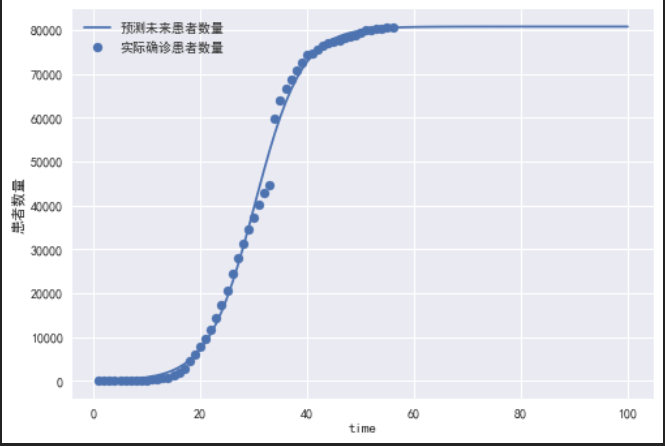# Gompertz增长模型
def func(p, t):
k, a, b = p
return k * a**(b**t)

08-08

#### Python Day---「抗击疫情」开发者在行动

02-1703-295388

#### 用 Python 预测新冠肺炎疫情发展（附源码）

02-074万+

#### 关于2019nCoV新冠肺炎的建模（Ⅱ）—基于Logistic模型的疫情预测

06-018332

#### 2020年东北三省数学建模联赛赛题

02-066478

#### python实现2019-nCoV疫情确诊数据拟合与预测

02-091230

#### 最新疫情数据报告已到达你的邮箱！

05-221630

#### 想做疫情分析却没有数据？看这篇就够了

02-251978

#### 肺炎疫情python数学模型预测

03-273350

#### 高手对2020年疫情趋势做一下数学模型分析

02-084788

#### python可视化来分析全国疫情©️2020 CSDN 皮肤主题: 创作都市 设计师: CSDN官方博客点击重新获取扫码支付1.余额是钱包充值的虚拟货币，按照1:1的比例进行支付金额的抵扣。
2.余额无法直接购买下载，可以购买VIP、C币套餐、付费专栏及课程。余额充值